# GNNs - 图神经网络学习笔记

## 0 链接

Graph Neural Networks: Models and Applications, AAAI 2020 Tutorial

## 1 研究背景

• 社交图谱；
• 传输图谱；
• 大脑图谱；
• 网页图谱；
• 分子图谱；
• 基因图谱。

• 链路预测；
• 节点分类；
• 社区检测；
• （网页）排序。

• 通过CNNs处理固定尺寸的图像/网格；
• 通过RNNs处理文本/序列。

• 各个节点的邻域节点数量各不相同；
• 图谱有着复杂的拓扑结构；
• 图谱也不存在固定的节点序列。

## 2 理论基础

### 2.1 基本图论

Basic Graph Theory

#### 2.1.1 图和图信号##### 图

$E = \{e_1, ... e_M\}$

$G = \{V, E\}$

##### 图信号

$f: V \to \mathbb{R}^{N \times d}$ 即，映射$$f$$把每一个节点$$v$$映射为一个$$d$$维实数向量： $v \to [f(1), f(2), ... f(d)]^T$

#### 2.1.2 图的矩阵表示

##### 邻接矩阵

$A = \begin{bmatrix} 0 & 1 & 0 & 0 & 0 & 0 & 0 & 0 \\ 1 & 0 & 1 & 0 & 0 & 1 & 0 & 0 \\ 0 & 1 & 0 & 1 & 0 & 1 & 1 & 0 \\ 0 & 0 & 1 & 0 & 1 & 0 & 0 & 0 \\ 0 & 0 & 0 & 1 & 0 & 1 & 0 & 0 \\ 0 & 1 & 1 & 0 & 1 & 0 & 1 & 0 \\ 0 & 0 & 1 & 0 & 0 & 1 & 0 & 1 \\ 0 & 0 & 0 & 0 & 0 & 0 & 1 & 0 \\ \end{bmatrix}$

##### 拉普拉斯矩阵

$L = \begin{bmatrix} 1 & -1 & 0 & 0 & 0 & 0 & 0 & 0 \\ -1 & 3 & -1 & 0 & 0 & -1 & 0 & 0 \\ 0 & -1 & 4 & -1 & 0 & -1 & -1 & 0 \\ 0 & 0 & -1 & 2 & -1 & 0 & 0 & 0 \\ 0 & 0 & 0 & -1 & 2 & -1 & 0 & 0 \\ 0 & -1 & -1 & 0 & -1 & 4 & -1 & 0 \\ 0 & 0 & -1 & 0 & 0 & -1 & 3 & -1 \\ 0 & 0 & 0 & 0 & 0 & 0 & -1 & 1 \\ \end{bmatrix}$

### 2.2 谱图理论

Spectral Graph Theory

$h(i) = \sum_{v_j \in \mathcal{N}(v_i)}(f(i) - f(j)) \in \mathbb{R}^d$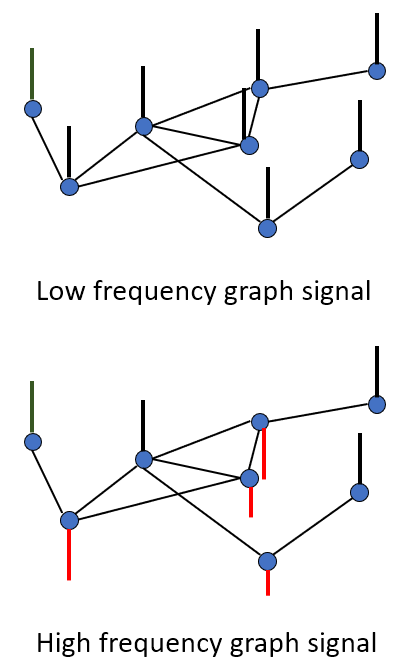• 低频和高频图谱信号；
• 低频图信号中，每个图节点的信号接近，意味着图信号变化平缓，即周期长，在频域中低频多，高频少；
• 高频图信号中，每个图节点的信号相差较大，意味着图信号变化剧烈、频繁，即周期短，在频域中，高频多，低频少。

• 一定有$$N$$个线性无关的特征向量；
• 特征值一定非负；
• 特征向量相互正交（特征向量组成的矩阵为正交矩阵）。

### 2.3 图傅里叶分析

Graph Fourier Analysis

#### 2.3.2 图傅里叶变换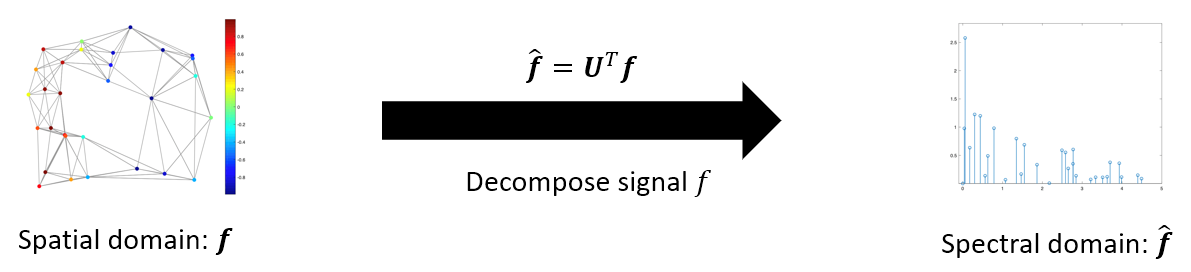#### 2.3.3 逆图傅里叶变换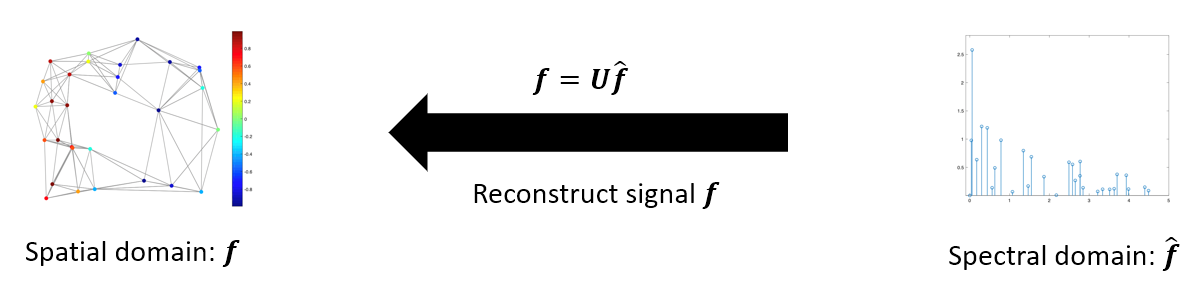## 3 模型

### 3.1 概述

1. 节点级（Node-level）：链路预测、节点分类等；
2. 图谱级（Graph-level）：图谱分类等。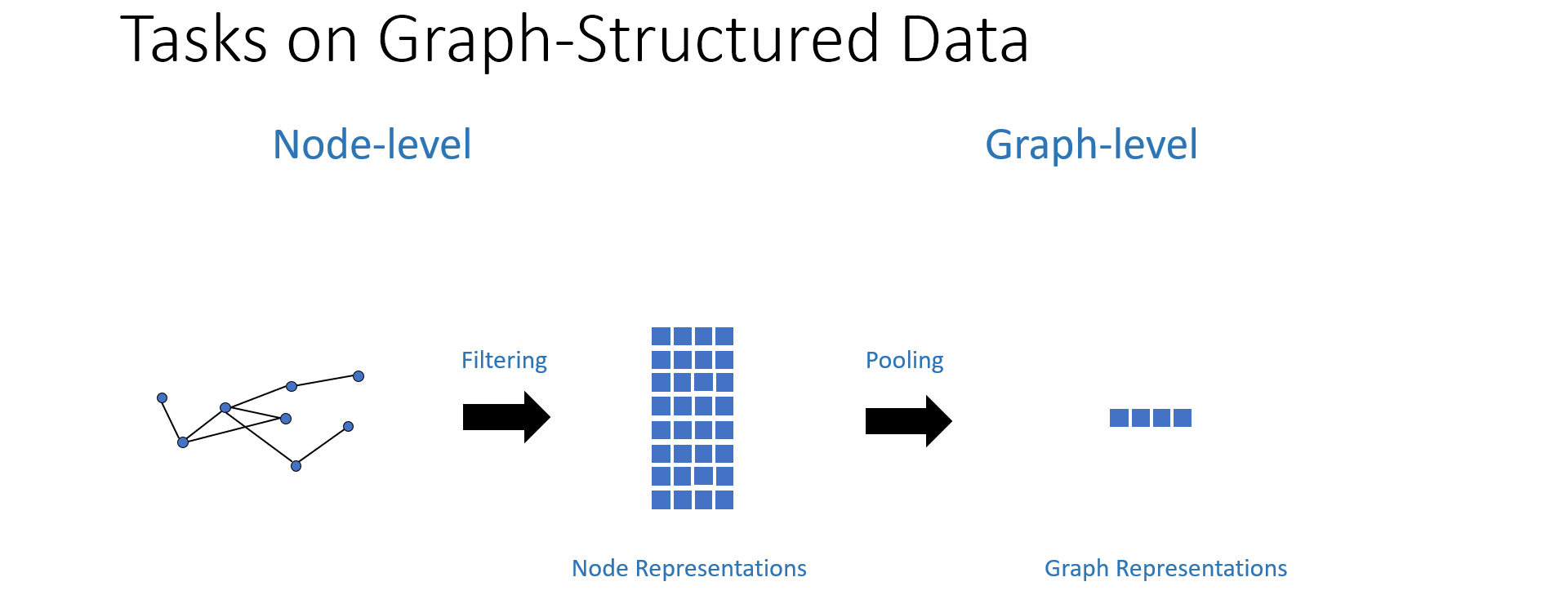1. 滤波（Filtering）：取得节点表征（Node Representation），用于解决节点级任务；
2. 池化（Pooling）：降维取得图谱表征（Graph Representation），用于解决图谱级任务。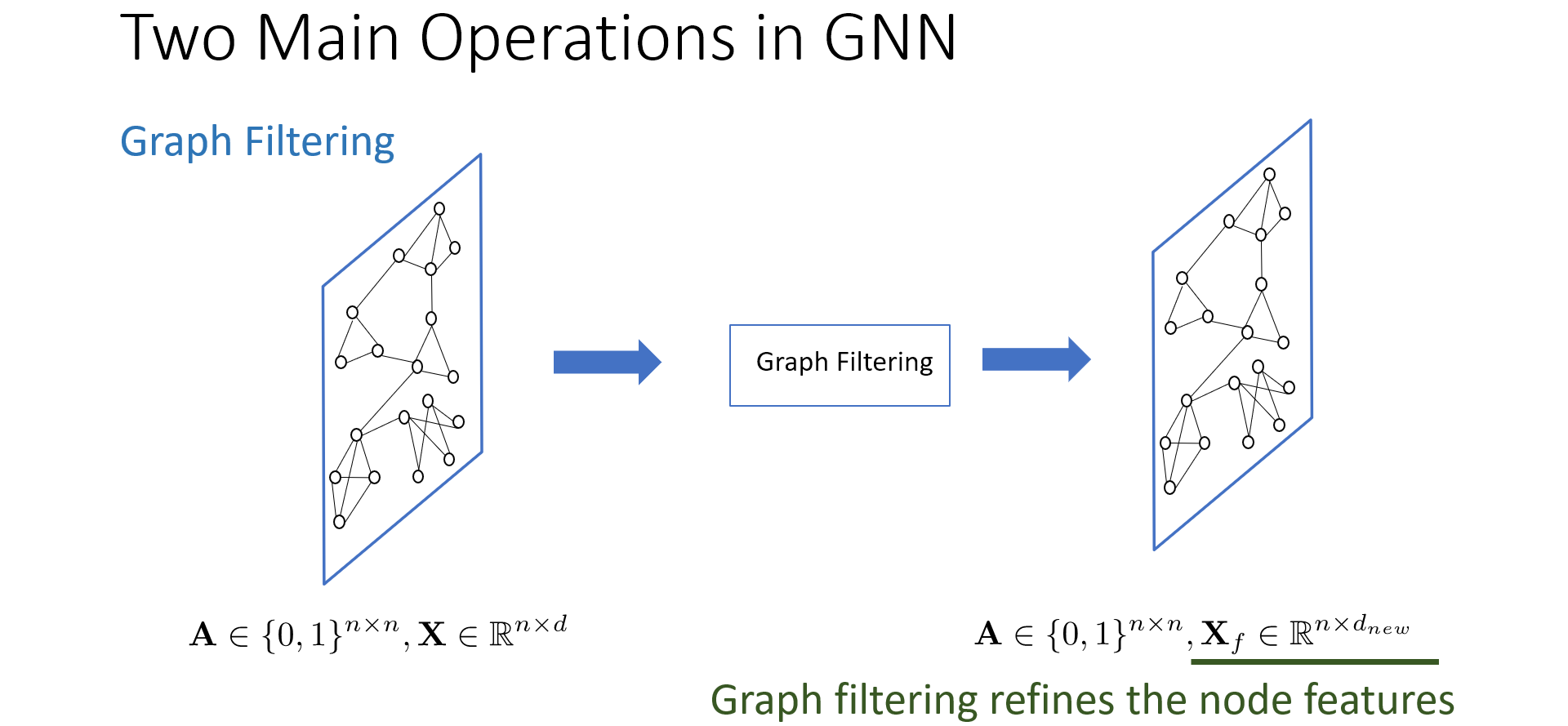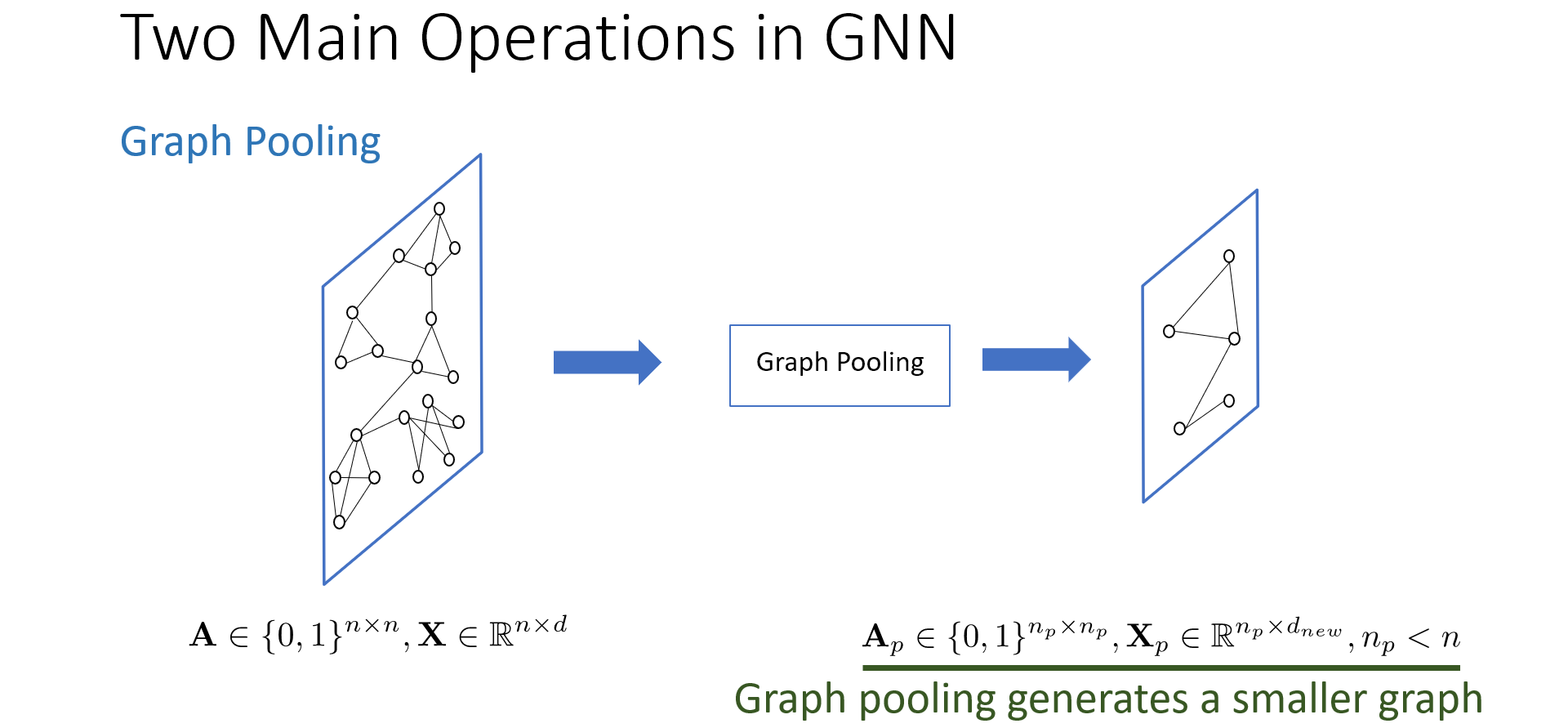### 3.2 GNN中的滤波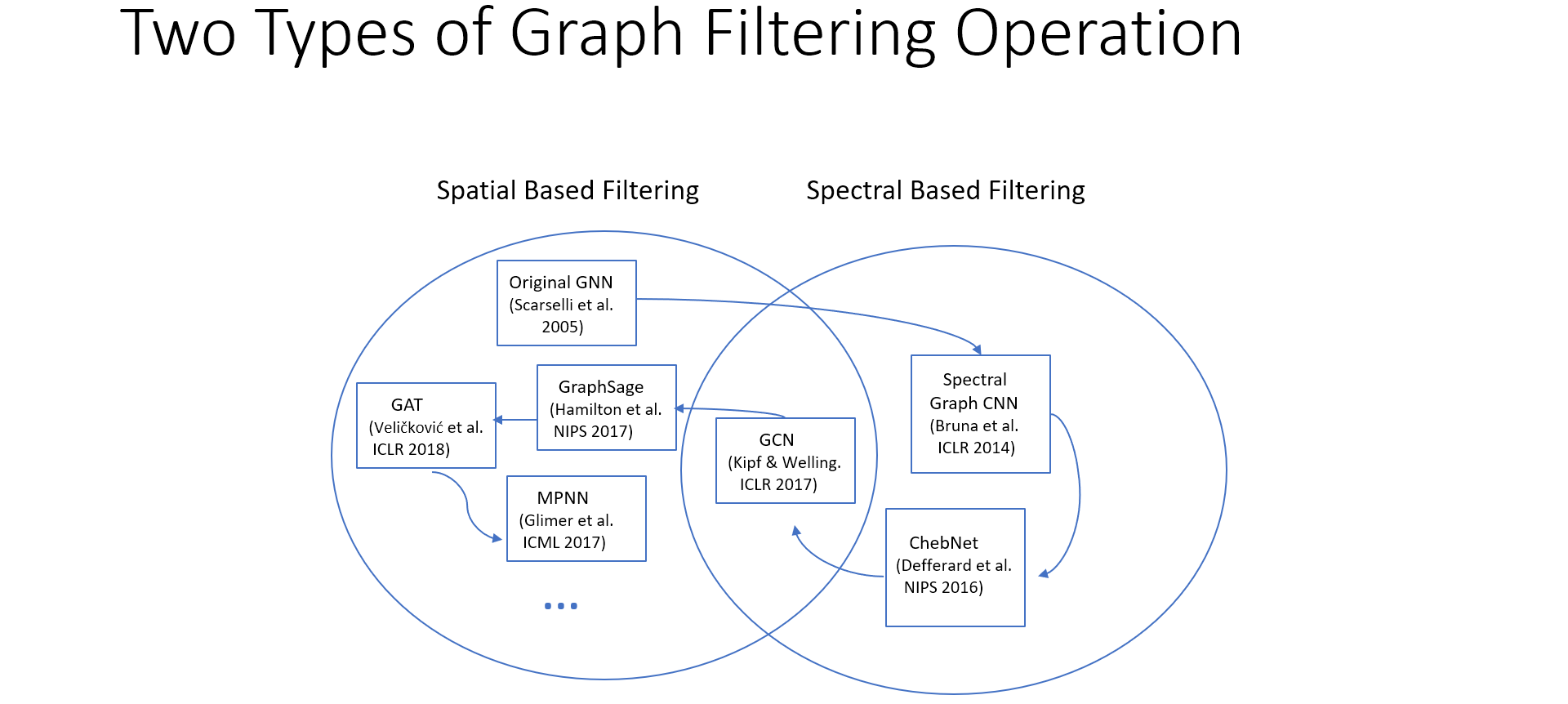• 频域GNN（Spectral-based GNN)
• 空域GNN（Spatial-based GNN

#### 3.2.1 频域滤波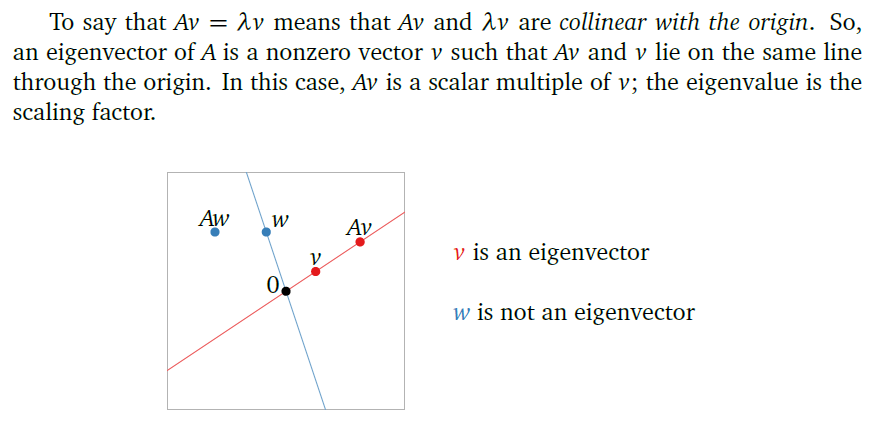1. 傅里叶变换：对信号 $$f$$ 进行傅里叶变换，取得相应于傅里叶模式 $$u_i$$ 的傅里叶系数 $$\hat{f_i} = u_i^T f$$ （或 $$\hat{f} = U^T f$$ ）；
2. 滤波：傅里叶变换得到了傅里叶模式 $$u_i$$ 的傅里叶系数 $$\hat{f_i}$$ ，那该傅里叶模式（即特征向量）是不是我们想要保留的呢？通过滤波，就可以根据该傅里叶模式（即特征向量）对应的频率（即特征值）进行滤波 $$\hat{g}(\lambda_i) u_i^T f$$（或$$\hat{g}(\Lambda) U^T f$$）；
3. 逆傅里叶变换：将滤波过的傅里叶系数 $$\hat{g}(\lambda_i) \hat{f_i}$$ 乘以对应的傅里叶分量（特征向量）$$u_i$$，逆傅里叶变换为滤波过的空域信号$$f_i^{(filtered)}$$（或$$f^{(filtered)}$$）。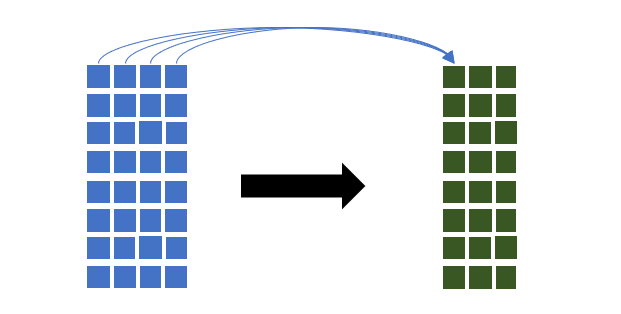#### 3.2.3 具体模型

##### 3.2.3.1 GNN

【空域滤波】

Scarselli F, Tsoi A C, Gori M, et al. Graphical-based learning environments for pattern recognition[C]//Joint IAPR International Workshops on Statistical Techniques in Pattern Recognition (SPR) and Structural and Syntactic Pattern Recognition (SSPR). Springer, Berlin, Heidelberg, 2004: 42-56.

Scarselli等人在后续的论文中又反复研究了图神经网络的理论及应用。

Scarselli F, Yong S L, Gori M, et al. Graph neural networks for ranking web pages[C]//The 2005 IEEE/WIC/ACM International Conference on Web Intelligence (WI'05). IEEE, 2005: 666-672.

Scarselli F, Gori M, Tsoi A C, et al. The graph neural network model[J]. IEEE Transactions on Neural Networks, 2008, 20(1): 61-80.

##### 3.2.3.2 Spectral Graph CNN

【频域滤波】

Bruna J, Zaremba W, Szlam A, et al. Spectral networks and locally connected networks on graphs[J]. arXiv preprint arXiv:1312.6203, 2013.

1.滤波函数近似效果差

2.滤波器完全依赖于训练时的图谱，无法迁移变通，每一个滤波值都是和对应节点绑定的；

3.模型参数规模庞大，因为一个图谱的节点数量$$N$$往往是很大的。

##### 3.2.3.3 ChebNet

【频域滤波】

Defferrard M, Bresson X, Vandergheynst P. Convolutional neural networks on graphs with fast localized spectral filtering[C]//Advances in neural information processing systems. 2016: 3844-3852.

ChebNet中，首先定义使用的拉普拉斯矩阵不再是基本的$$L=D-A$$，而是规格化（normalized）拉普拉斯矩阵，即：$$L = I_N - D^{-1/2} A D^{-1/2}$$

Hammond D K, Vandergheynst P, Gribonval R. Wavelets on graphs via spectral graph theory[J]. Applied and Computational Harmonic Analysis, 2011, 30(2): 129-150.

Geddes K O. Near-minimax polynomial approximation in an elliptical region[J]. SIAM Journal on Numerical Analysis, 1978, 15(6): 1225-1233.

$$f(x)$$目标函数的$$n+2$$个采样点$$[x_1, x_2, \dots, x_{n+2}]$$进行近似时，极小极大近似算法（minimax approximation algorithm），典型代表如：Remez algorithmRemez exchange algorithm可以使得$$n$$阶多项式$$P_n(x)=a_0 + a_1 x + a_2 x^2 + \dots + a_n x^n$$对目标函数实现minimax近似（最小化最大误差，使$$n+2$$个采样点的最大误差最小化），形式化为，解线性方程系统： $a_0 + a_1 x_i + a_2 x_i^2 + \dots + a_n x_i^n + (-1)^i E = f(x)\qquad(where\quad i=1,2,\dots,n+2)$ 其中$a_0, a_1, \dots, a_n$是多项式$P_n(x)$的多项式系数。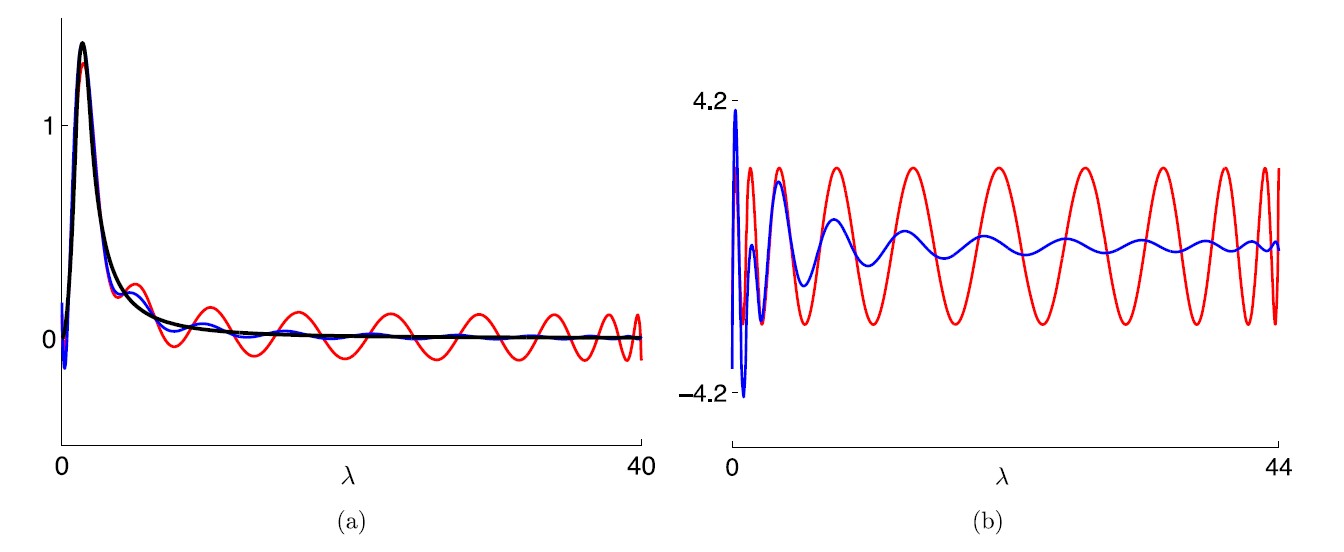• 左图(a)中，黑色表示目标函数，为小波核函数$$g(\lambda)$$，蓝色表示截断切比雪夫展开式（truncated Chebyshev expansion），红色表示minimax多项式近似。
• 右图(b)中，表示的是两个近似函数，即截断切比雪夫展开式和minimax多项式，与目标函数的近似误差。

##### 3.2.3.4 GCN

【频域滤波】

Kipf T N, Welling M. Semi-supervised classification with graph convolutional networks[J]. arXiv preprint arXiv:1609.02907, 2016.

Graph Convolutional Network (GCN)

GCN将切比雪夫展开式限制为1阶，即$$K=1$$，则滤波器函数表示为： $g(\lambda) = \sum_{k=0}^1 \theta_k T_k(\lambda) = \theta_0 T_0(\lambda) + \theta_1 T_1(\lambda) = \theta_0 + \theta_1 \lambda$ 对于一阶切比雪夫多项式，GCN还增加了限制条件，限定$$\theta = \theta_0 = - \theta_1$$，使参数只剩$$\theta \in \mathbb{R}$$一项。滤波器函数表示为： $g(\lambda) = \theta - \theta \lambda = \theta(1 - \lambda)$ 简化2：特征值约束

GCN将特征值的最大值限定为2，即$$\lambda_{max} = 2$$

GCN可以用于多通道的信号处理，对于从$$d_1$$个通道的输入信号到$$d_2$$个通道的输出信号的滤波映射，GCN的一层可形式化为以下矩阵形式： $Z = \tilde{D}^{-1/2} \tilde{A} \tilde{D}^{-1/2} X \Theta$

• $$X \in \mathbb{R}^{N \times d_1}$$表示频域滤波前的输入信号；
• $$\Theta \in \mathbb{R}^{d_1 \times d_2}$$表示$$d_1 \times d_2$$个滤波的参数；
• $$Z \in \mathbb{R}^{N \times d_2}$$表示频域滤波后的输出信号。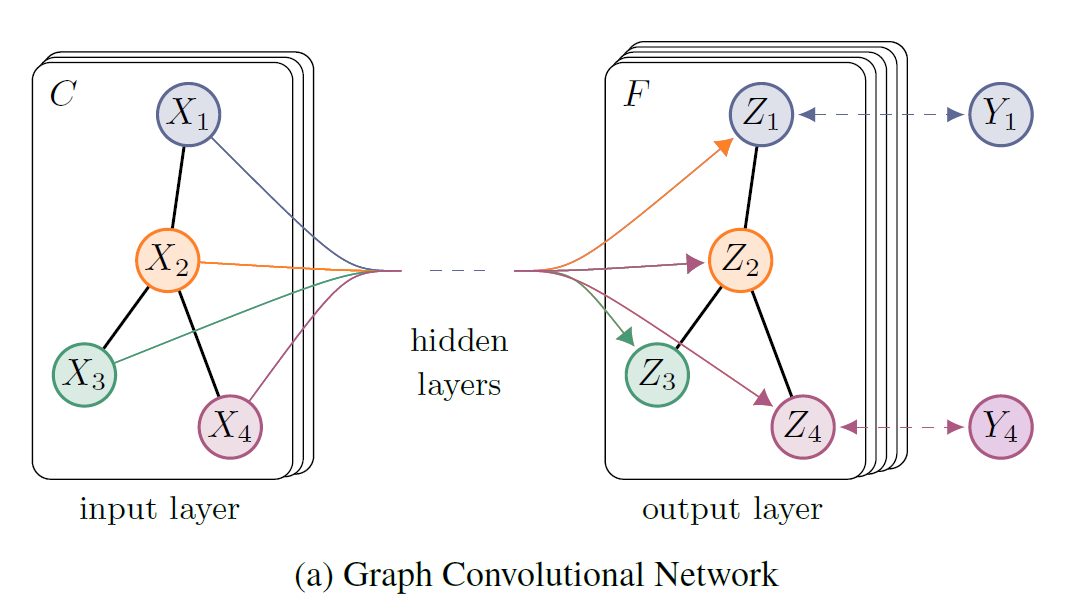GCN可以用于实现半监督学习，即，以有标签的节点来计算损失，进行训练。具体的，论文中基于有标签节点计算交叉熵损失： $\mathcal{L} = - \sum_{l \in \mathcal{Y}_L} \sum_{f=1}^F Y_{lf} \ln Z_{lf}$ 其中，$$\mathcal{Y}_L$$是有标签的节点序号集合。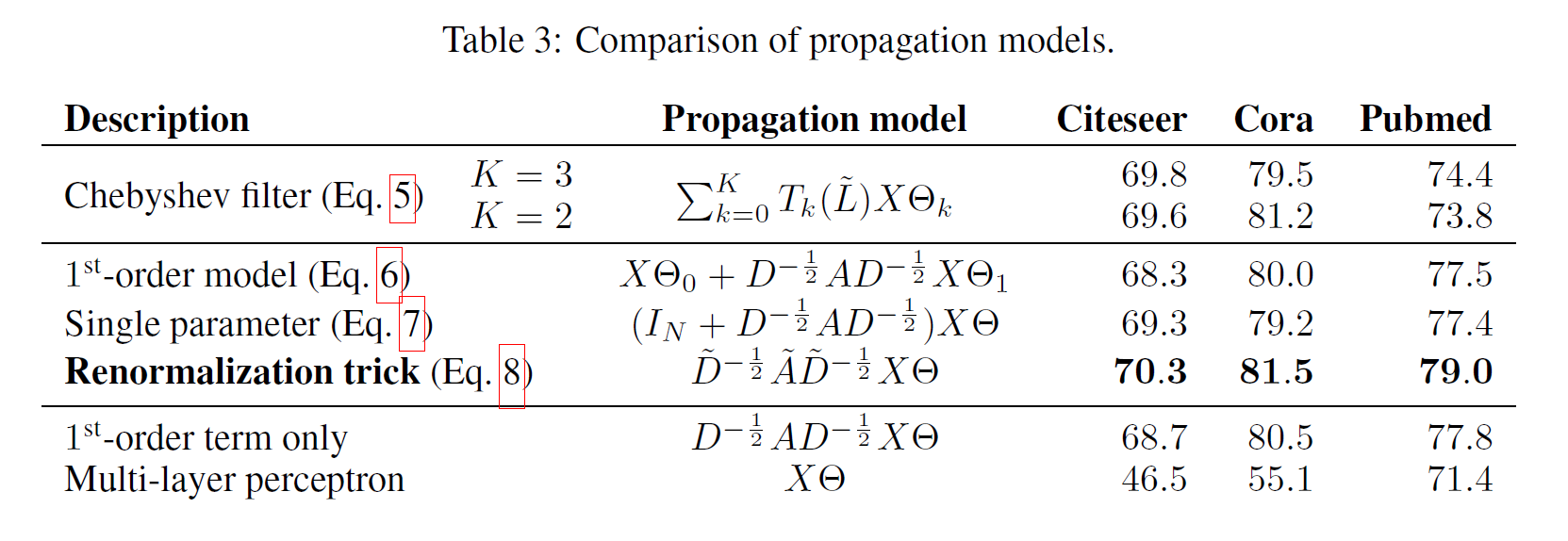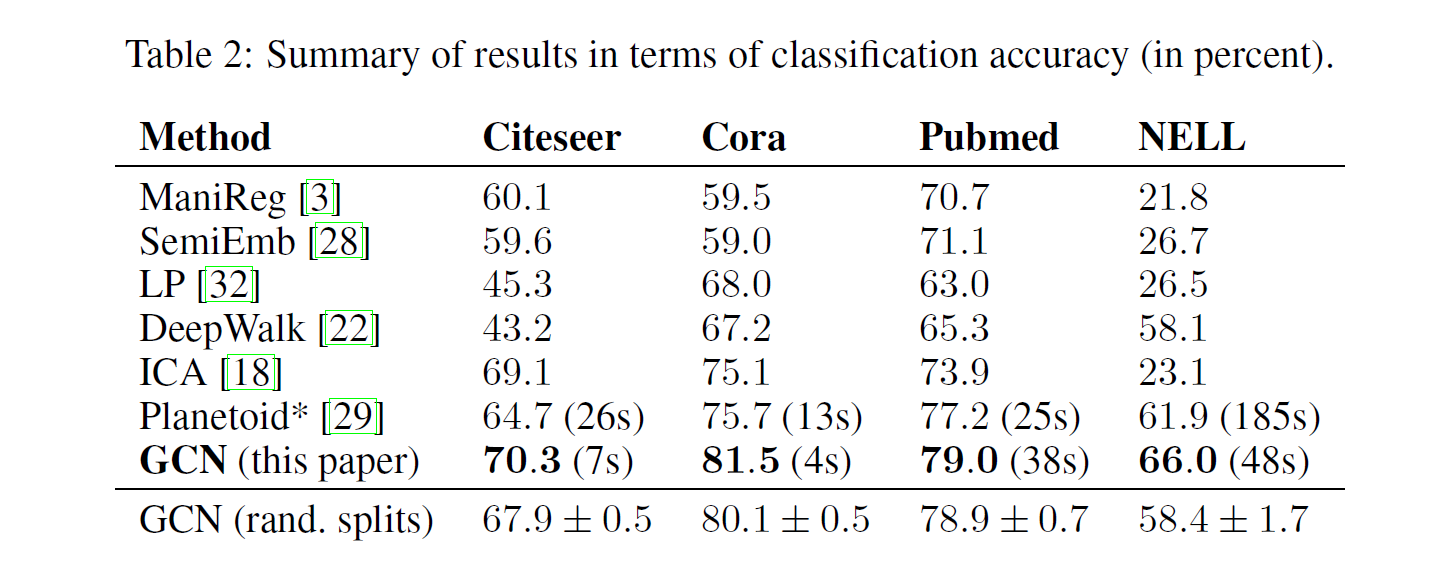### 3.4 GNN的鲁棒性

Robustness of GNN

### 3.5 GNN的扩展学习

Scalable Learning for GNN

## 4 应用

### 4.3 推荐系统微信支付宝PayPal